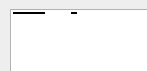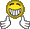# Draw rectangle is somewhat slow, when it draws the house for the test file?ClassicListThreaded15 messagesOpen this post in threaded view
|

## Draw rectangle is somewhat slow, when it draws the house for the test file?

 My program draws the graphics for the test file as the expected one. But when it draws a rectangle, like for the window and the door, It is somewhat slow(but I used the optimized way of drawing a line). My assumption for it being slow was, I've only drawLine method which draws vertical, horizontal and diagonal lines, so to check all those conditions I've one big condition inside the while loop, that selects the correct condition for the current input values(like a >= dx or a <= dx), and that condition is executed in every iteration. But I can only check the condition only one time, so that I can get the correct condition for the while loop. What I want to do         let xCondition = (~(a > dx));         let yCondition = (~(b > dy));         if(dx < 0) {             let xCondition = (~(a < dx));         }         if(dy < 0){             let yCondition = (~(b < dy));         } while(xCondition & yCondition) {} But this one is not working because of refactoring the conditions. When I didn't refactor the condition inside the while loop, it works fine. But this one makes my program slow I think.         if(dx < 0) {             let xDirection = -1;         }         if(dy < 0){             let yDirection = -1;         }         while((((xDirection = 1) & ~(a > dx)) | ((xDirection = -1) & ~(a < dx))) & (((yDirection = 1) & ~(b > dy)) | ((yDirection = -1) & ~(b < dy)))) { }
Open this post in threaded view
|

## Re: Draw rectangle is somewhat slow, when it draws the house for the test file?

 If you have a single function for drawing any kind of line, it might be slow. Especially if it draws the line one pixel at a time. There is one kind of line, which can be drawn very fast compared to the others. And this line drawing can also be used in the drawRectangle, drawCircle, etc, and even in the general drawLine.
Open this post in threaded view
|

## Re: Draw rectangle is somewhat slow, when it draws the house for the test file?

 Okay Ivant, now I refactored into drawHorizontal, drawVertical and drawDiagonal functions. And It is not bad now, so how can I draw line without drawing pixel by pixel like for the horizontal one?
Open this post in threaded view
|

## Re: Draw rectangle is somewhat slow, when it draws the house for the test file?

 You can't always do so, but if the line's slope is less than 45 degrees, it will consist of small horizontal lines. The closer to horizontal the line is, the bigger the effect.
Open this post in threaded view
|

## Re: Draw rectangle is somewhat slow, when it draws the house for the test file?

 So is there any other way drawing a horizontal line without drawing pixel by pixel?
Open this post in threaded view
|

## Re: Draw rectangle is somewhat slow, when it draws the house for the test file?

 Yes, there is. What are the steps to write a single pixel on the screen?
Open this post in threaded view
|

## Re: Draw rectangle is somewhat slow, when it draws the house for the test file?

 1. Find the physical memory location of the given x, y in terms of bytes. 2. Then find the mask for actual location of the bit we want to set. 3. Then depending on the color, which is currently selected we may "or" or "and" with the mask so as to change the bit value to the selected color.
Open this post in threaded view
|

## Re: Draw rectangle is somewhat slow, when it draws the house for the test file?

 Well, your 3rd step is actually more like 3 separate steps: 3a. read the word 3b. change the bit 3c. write the changed word back In horizontal line, lots of pixels are in the same word and they are also next to each other. Let's use the underscore _ for pixels that are not part of the line, so we don't want to change them, and X for pixels that we want to change. Basically, you have 4 cases: 1: ______XXXXXX (some _, rest are X total 16 - left part of the line) 2. XXXX_____ (right part of the line) 3. XXXXXXXX (16 X - middle part of the line) 4. ___XXX___ (the whole line is shorter than the word) You can construct bit masks to change several pixels at the same time. And for the 3rd case, you don't even need to read the previous value on this address, because you are replacing it all.
Open this post in threaded view
|

## Re: Draw rectangle is somewhat slow, when it draws the house for the test file?

 Great Ivant. Yeah I was thinking when you asked me the steps for drawing a pixel? If you can please give me the idea for constructing bit masks to change several pixels at a time?
Open this post in threaded view
|

## Re: Draw rectangle is somewhat slow, when it draws the house for the test file?

 Well, you look at the first pattern, how many bit masks would you need? If they aren't too many, perhaps it makes sense to pre-compute them in Screen.init. The bit-patterns for the second case seem to be easily computable from the ones we already have (mostly inverting them). The third case doesn't need any. So the only remaining is the fourth case. Now is there a fast and easy way to compute the pattern using the ones we already have?
Open this post in threaded view
|

## Re: Draw rectangle is somewhat slow, when it draws the house for the test file?

 Thank you Ivant, you helped me a lot. Since we handle all lines in a word, XXXXXX, in its own we only need 15 bit mask. And I precompute it in Screen.init. The fourth case is by AND, the first and the second case. And now my program draws fast. But the output is not as expected. Like drawing from 0 to 18 px is not correct. do Screen.drawLine(0,0, 18,0); This program should set the first word all 1 and in the second word the first 3 bits. And my program handles those cases. And I even print memory locations at 16384 = -1, 16384 = -8192  and 16384 = 0; -1 = 1111111111111111 -8192 = 1110000000000000 And this seems correct, but screen draws not correctly.Open this post in threaded view
|

## Re: Draw rectangle is somewhat slow, when it draws the house for the test file?

 It looks like you are using the "inverted" maps, in the sense that you are drawing from the wrong end. That is, instead of -8192, you should write 7 (0000000000000111). It is a bit confusing, but that's how the HACK computer works. An easy way to test this is to start the CPUEmulator and manually change the contents of the addresses.
Open this post in threaded view
|

## Re: Draw rectangle is somewhat slow, when it draws the house for the test file?

 Make sense, but I don't have any idea now, to make the change .
 Finally it is working correctly. Thank you so much Ivant. Thank you all.